Tamilnadu State Board New Syllabus Samacheer Kalvi 12th Business Maths Guide Pdf Chapter 4 Differential Equations Ex 4.6 Text Book Back Questions and Answers, Notes.

## Tamilnadu Samacheer Kalvi 12th Business Maths Solutions Chapter 4 Differential Equations Ex 4.6

Choose the most suitable answer from the given four alternatives:

Question 1.
The degree of the differential equation
$$\frac { d^2y }{dx^4}$$ – ($$\frac { d^2y }{dx^2}$$) + $$\frac { dy }{dx}$$ = 3
(a) 1
(b) 2
(c) 3
(d) 4
Solution:
(a) 1
Hint:
Since the power of $$\frac{d^{4} y}{d x^{4}}$$ is 1Question 2.
The order and degree of the differential equation $$\sqrt{\frac { d^2y }{dx^2}}$$ = $$\sqrt{\frac { dy }{dx}+5}$$ are respectively.
(a) 2 and 2
(b) 3 and 2
(c) 2 and 1
(d) 2 and 3
Solution:
(a) 1
Hint:
Squaring on both sides
$$\frac { d^2y }{dx^2}$$ = $$\frac { dy }{dx}$$ + 5
Highest order derivative is $$\frac { d^2y }{dx^2}$$
∴ order = 2
Power of the highest order derivative $$\frac { d^2y }{dx^2}$$ = 1
∴ degree = 1

Question 3.
The order and degree of the differential equation
($$\frac { d^2y }{dx^2}$$)3/2 – $$\sqrt{(\frac { dy }{dx})}$$ – 4 = 0
(a) 2 and 6
(b) 3 and 6
(c) 1 and 4
(d) 2 and 4
Solution:
(a) 2 and 6
Hint: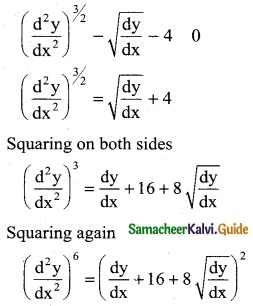Highest order derivative is $$\frac { d^2y }{dx^2}$$
∴ Order = 2
Power of the highest order derivative $$\frac { d^2y }{dx^2}$$ is
∴ degree = 6Question 4.
The differential equation ($$\frac { dx }{dy}$$)³ + 2y1/2 = x
(a) of order 2 and degree 1
(b) of order 1 and degree 3
(c) of order 1 and degree 6
(d) of order 1 and degree 2
Solution:
(b) of order 1 and degree 3
Hint: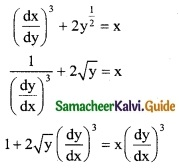Highest order derivative is $$\frac { dy }{dx}$$
∴ order = 1
Power of the highest order derivative $$\frac { dy }{dx}$$ is 3
∴ degree = 3

Question 5.
The differential equation formed by eliminating a and b from y = aex + be-x
(a) $$\frac { d^2y }{dx^2}$$ – y = 0
(b) $$\frac { d^2y }{dx^2}$$ – $$\frac { dy }{dx}$$y = 0
(c) $$\frac { d^2y }{dx^2}$$ = 0
(d) $$\frac { d^2y }{dx^2}$$ – x = 0
Solution:
(a) $$\frac { d^2y }{dx^2}$$ – y = 0
Hint: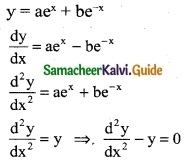Question 6.
If y = ex + c – c³ then its differential equation is
(a) y = x$$\frac { dy }{dx}$$ + $$\frac { dy }{dx}$$ – ($$\frac { dy }{dx}$$)³
(b) y + ($$\frac { dy }{dx}$$)³ = x $$\frac { dy }{dx}$$ – $$\frac { dy }{dx}$$
(c) $$\frac { dy }{dx}$$ + ($$\frac { dy }{dx}$$)³ – x$$\frac { dy }{dx}$$
(d) $$\frac { d^3y }{dx^3}$$ = 0
Solution:
(a) y = x$$\frac { dy }{dx}$$ + $$\frac { dy }{dx}$$ – ($$\frac { dy }{dx}$$)³
Hint:
y = cx + c – c³ ……… (1)
$$\frac { dy }{dx}$$ = c
(1) ⇒ y = x$$\frac { dy }{dx}$$ + $$\frac { dy }{dx}$$ – ($$\frac { dy }{dx}$$)³

Question 7.
The integrating factor of the differential equation $$\frac { dy }{dx}$$ + Px = Q is
(a) e∫pdx
(b) ePdx
(c) ePdy
(d) e∫pdy
Solution:
(d) e∫pdyQuestion 8.
The complementary function of (D² + 4) y = e2x is
(a) (Ax+B)e2x
(b) (Ax+B)e-2x
(c) A cos2x + B sin2x
(d) Ae-2x + Be2x
Solution:
(c) A cos2x + B sin2x
Hint:
A.E = m2 + 4 = 0 ⇒ m = ±2i
C.F = e0x (A cos 2x + B sin 2x)

Question 9.
The differential equation of y = mx + c is (m and c are arbitrary constants)
(a) $$\frac { d^2y }{dx^2}$$ = 0
(b) y = x$$\frac { dy }{dx}$$ + o
(c) xdy + ydx = 0
(c) ydx – xdy = 0
Solution:
(a) $$\frac { d^2y }{dx^2}$$ = 0
Hint:
y = mx + c
$$\frac { dy }{dx}$$ = m ⇒ $$\frac { d^2y }{dx^2}$$ = 0Question 10.
The particular intergral of the differential equation $$\frac { d^2y }{dx^2}$$ – 8$$\frac { dy }{dx}$$ + 16y = 2e4x
(a) $$\frac { x^2e^{4x} }{2!}$$
(b) y = x$$\frac { e^{4x} }{2!}$$
(c) x²e4x
(d) xe4x
Solution:
(c) x²e4x
Hint: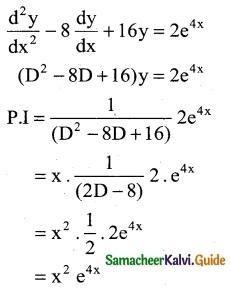Question 11.
Solution of $$\frac { dx }{dy}$$ + Px = 0
(a) x = cepy
(b) x = ce-py
(c) x = py + c
(d) x = cy
Solution:
(b) x = ce-pyQuestion 12.
If sec2x x isa na intergranting factor of the differential equation $$\frac { dx }{dy}$$ + Px = Q then P =
(a) 2 tan x
(b) sec x
(c) cos 2 x
(d) tan 2 x
Solution:
(a) 2 tan x
Hint:
I.F = sec² x
e∫pdx = sec²x
∫pdx = log sec² x
∫pdx = 2 log sec x
∫pdx = 2∫tan x dx ⇒ p = 2 tan x

Question 13.
The integrating factor of the differential equation is x $$\frac { dy }{dx}$$ – y = x²
(a) $$\frac { -1 }{x}$$
(b) $$\frac { 1 }{x}$$
(c) log x
(c) x
Solution:
(b) $$\frac { 1 }{x}$$
Hint: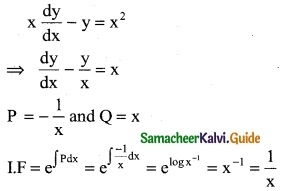Question 14.
The solution of the differential equation where P and Q are the function of x is
(a) y = ∫Q e∫pdx dx + c
(b) y = ∫Q e-∫pdx dx + c
(c) ye∫pdx = ∫Q e∫pdx dx + c
(c) ye∫pdx = ∫Q e-∫pdx dx + c
Solution:
(c) ye∫pdx = ∫Q e∫pdx dx + c

Question 15.
The differential equation formed by eliminating A and B from y = e-2x (A cos x + B sin x) is
(a) y2 – 4y1 + 5 = 0
(b) y2 + 4y – 5 = 0
(c) y2 – 4y1 + 5 = 0
(d) y2 + 4y1 – 5 = 0
Solution:
(d) y2 + 4y1 – 5 = 0
Hint:
y = e-2x (A cosx + B sinx)
y e2x = A cosx + B sinx ………. (1)
y(e2x) (2) + e2x $$\frac { dy }{dx}$$ = A(-sin x) + B cos x ………. (2)
Differentiating w.r.to x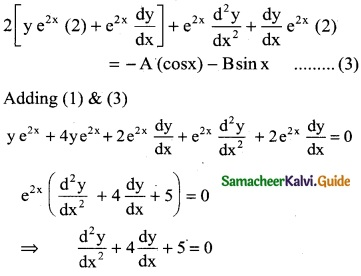Question 16.
The particular integral of the differential equation f (D) y = eax where f(D) = (D – a)²
(a) $$\frac { x^2 }{2}$$ eax
(b) xeax
(c) $$\frac { x }{2}$$ eax
(d) x² eax
Solution:
(a) $$\frac { x^2 }{2}$$ eax

Question 17.
The differential equation of x² + y² = a²
(a) xdy + ydx = 0
(b) ydx – xdy = 0
(c) xdx – ydx = 0
(d) xdx + ydy = 0
Solution:
(d) xdx + ydy = 0
Hint:
x2 + y2 = a2
⇒ 2x + 2y $$\frac{d y}{d x}$$ = 0
⇒ x dx + y dy = 0Question 18.
The complementary function of $$\frac { d^y }{dx^2}$$ – $$\frac { dy }{dx}$$ = 0 is
(a) A + Bex
(b) (A + B)ex
(c) (Ax + B)ex
(d) Aex + B
Solution:
(a) A + Bex
Hint:
A.E is m2 – m = 0
⇒ m(m – 1) = 0
⇒ m = 0, 1
CF is Ae0x + Bex = A + Bex

Question 19.
The P.I of (3D² + D – 14) y = 13e2x is
(a) $$\frac { 1 }{2}$$ ex
(b) xe2x
(c) $$\frac { x^2 }{2}$$ e2x
(d) Aex + B
Solution:
(b) xe2x
Hint:
(3D² + D – 14) y = 13e2x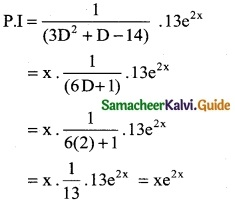Question 20.
The general solution of the differential equation $$\frac { dy }{dx}$$ = cos x is
(a) y = sinx + 1
(b) y = sinx – 2
(c) y = cosx + C, C is an arbitary constant
(d) y = sinx + C, C is an arbitary constant
Solution:
(d) y = sinx + C, C is an arbitary constant
Hint:
$$\frac { dy }{dx}$$ = cos x
dy = cos x dx
∫dy = ∫cos x dx ⇒ y = sin x + c

Question 21.
A homogeneous differential equation of the form $$\frac { dy }{dx}$$ = f($$\frac { y }{x}$$) can be solved by making substitution.
(a) y = v x
(b) y = y x
(c) x = v y
(d) x = v
Solution:
(a) y = v xQuestion 22.
A homogeneous differential equation of the form $$\frac { dy }{dx}$$ = f($$\frac { x }{y}$$) can be solved by making substitution.
(a) x = v y
(b) y = v x
(c) y = v
(d) x = v
Solution:
(a) y = v x

Question 23.
The variable separable form of $$\frac { dy }{dx}$$ = $$\frac { y(x-y) }{x(x+y)}$$ by taking y = v x and $$\frac { dy }{dx}$$ = v + x $$\frac { dy }{dx}$$
(a) $$\frac { 2v^2 }{1+v}$$ dv = $$\frac { dx }{x}$$
(b) $$\frac { 2v^2 }{1+v}$$ dv = –$$\frac { dx }{x}$$
(c) $$\frac { 2v^2 }{1-v}$$ dv = $$\frac { dx }{x}$$
(d) $$\frac { 1+v }{2v^2}$$ dv = –$$\frac { dx }{x}$$
Solution:
(d) $$\frac { 1+v }{2v^2}$$ dv = –$$\frac { dx }{x}$$
Hint: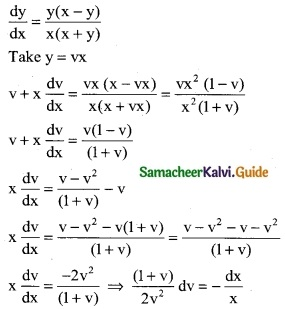Question 24.
Which of the following is the homogeneous differential equation?
(a) (3x – 5) dx = (4y – 1) dy
(b) xy dx – (x³ + y³) dy = 0
(c) y²dx + (x² – xy – y²) dy = 0
(d) (x² + y) dx (y² + x) dy
Solution:
(c) y²dx + (x² – xy – y²) dy = 0

Question 25.
The solution of the differential equation $$\frac { dy }{dx}$$ = $$\frac { y }{x}$$ + $$\frac { f(\frac { y }{x}) }{ f(\frac { y }{x}) }$$ is
(a) f$$\frac { y }{x}$$ = k x
(b) x f$$\frac { y }{x}$$ = k
(c) f$$\frac { y }{x}$$ = k y
(d) x f$$\frac { y }{x}$$ = k
Solution:
(a) f$$\frac { y }{x}$$ = k x